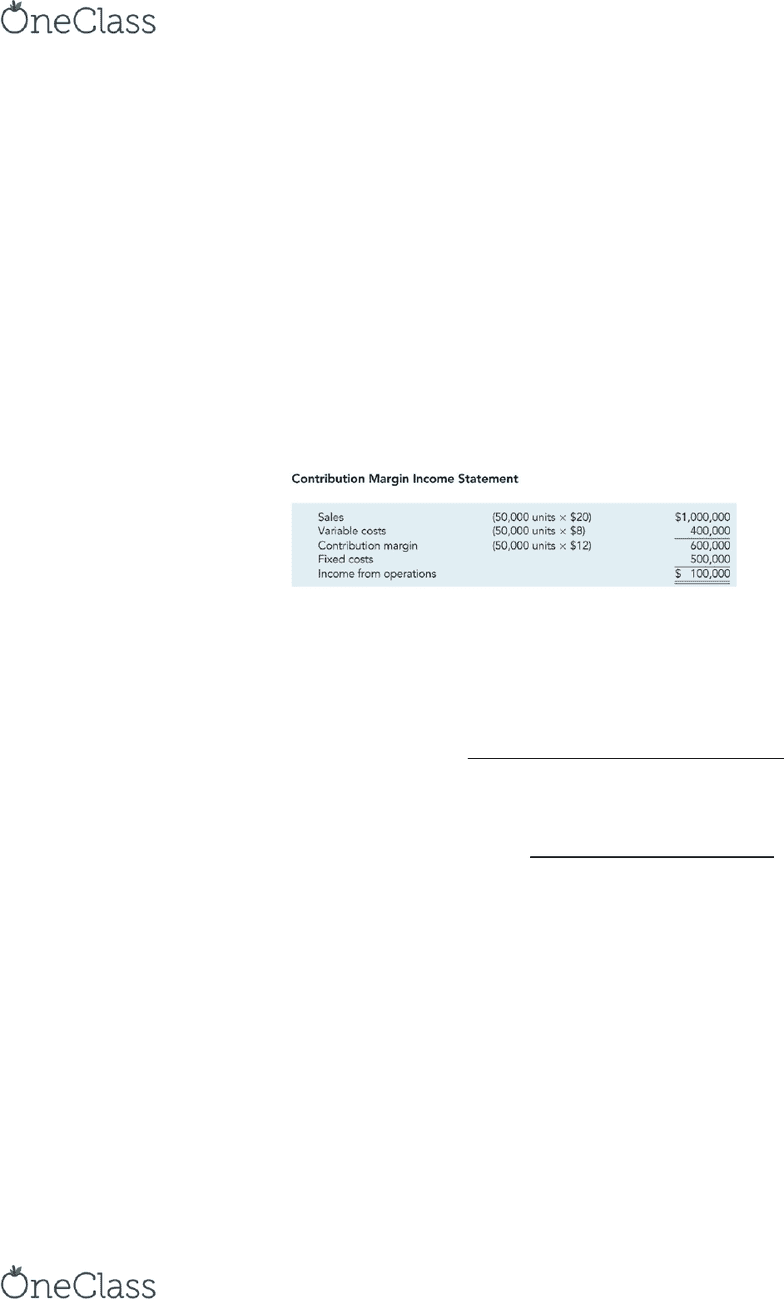Class Notes (1,100,000)
CA (620,000)
Ryerson (30,000)
ACC 406 (200)
Lecture 4

# ACC 406 Lecture Notes - Lecture 4: Operating Leverage, Earnings Before Interest And Taxes, Fixed Cost

Department
Accounting
Course Code
ACC 406
Professor
Alison Beavis
Lecture
4

This preview shows half of the first page. to view the full 3 pages of the document.Cost-Volume-Profit Analysis: A Managerial Planning Tool
- This powerful tool for planning and decision making can be used to calculate:
o The number of units that must be sold to break even
o The impact of a given reduction in fixed costs on the break-even point
o The impact of an increase in price on profit
Break-Even Point
- Where profits are zero
- Sales Variable Cost = Contribution Margin
- Contribution Margin Fixed Costs = Operating Income
- Contribution Margin Ratio = Contribution Margin/Sales
- Operating income = sales total variable expenses total fixed expenses
Break-even number of units = total fixed expenses/ price variable cost
Variable cost ratio= variable cost per unit/price
Break-even sales= total fixed expenses/contribution margin ratio
Units to be Sold to Achieve a Target Income
     
  
Sales Revenue to Achieve Target Income
     

Profit-Volume Graph
- Visually portrays the relationship between profits and units sold
- Operating income is the dependent variable
- Units sold is the independent variable
- The profit-volume graph is the graph of the operating income equation
find more resources at oneclass.com
find more resources at oneclass.com
###### You're Reading a Preview

Unlock to view full version